Fifth Chapter Lesson-6 Conditional Control Statement Related algorithm & flowchart.

At the end of this lesson-

• 1. You will be able to write algorithm & flowchart for determining an integer number is whether even or odd.
• 2. You will be able to write algorithm & flowchart for determining a number is whether positive or negative.
• 3. You will be able to write algorithm & flowchart for determining a year is whether leap year or not leap year.
• 4. You will be able to write algorithm & flowchart for determining larger number between two numbers.
• 5. You will be able to write algorithm & flowchart for determining LCM of two integer numbers.
• 6. You will be able to write algorithm & flowchart for determining GCD of two integer numbers.
• 7. You will be able to write algorithm & flowchart for determining the smallest number among three numbers.
• 8. You will be able to write algorithm & flowchart for determining the largest number among three numbers.

1. Algorithm & flowchart for determining an integer number is whether even or odd.

• Algorithm
• Step-1: Start
• Step-2: Input a number in variable n
• Step-3: r = n mod 2
• Step-4: If r=0, then print the number even and go to step-6,
•             Otherwise go to step-5
• Step-5: Print the number odd
• Step-6: Stop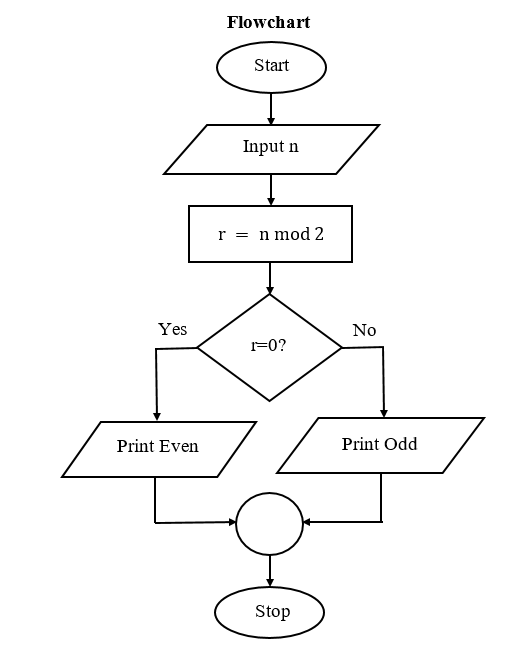2. Algorithm & flowchart for determining a number is whether positive or negative.

• Algorithm
• Step-1: Start
• Step-2: Input a number in variable n
• Step-3: If (n≤0), then print the number positive and go to step-5,
•             Otherwise go to step-4
• Step-4: Print the number negative
• Step-5: Stop3. Algorithm & flowchart for determining a year is whether leap year or not leap year.

• Algorithm
• Step-1: Start
• Step-2: Input a year in variable y
• Step-3: If ((y mod 400 = 0) OR ((y mod 100 ≠ 0) AND (y mod 4 = 0))), then go to step-4,
•             Otherwise go to step-5
• Step-4: Print leap year and go to step-6
• Step-5: Print not leap year
• Step-6: Stop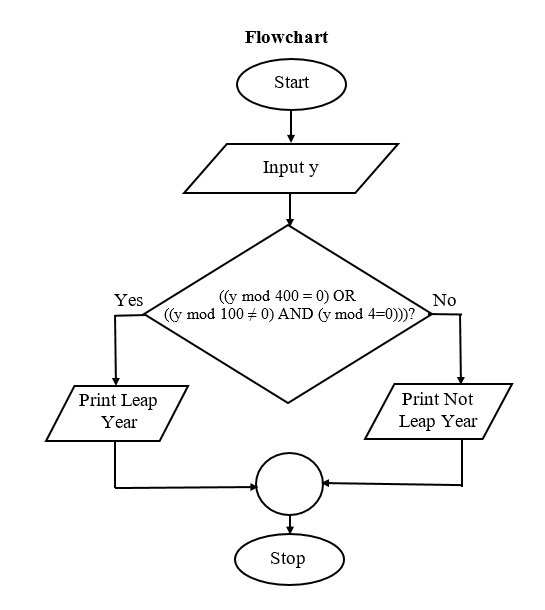4. Algorithm & flowchart for determining larger number between two numbers.

• Algorithm
• Step-1: Start
• Step-2: Input two numbers in variable a and b
• Step-3: If (a>b), then print value of a and go to step-5,
•             Otherwise go to step-4
• Step-4: Print value of b
• Step-5: Stop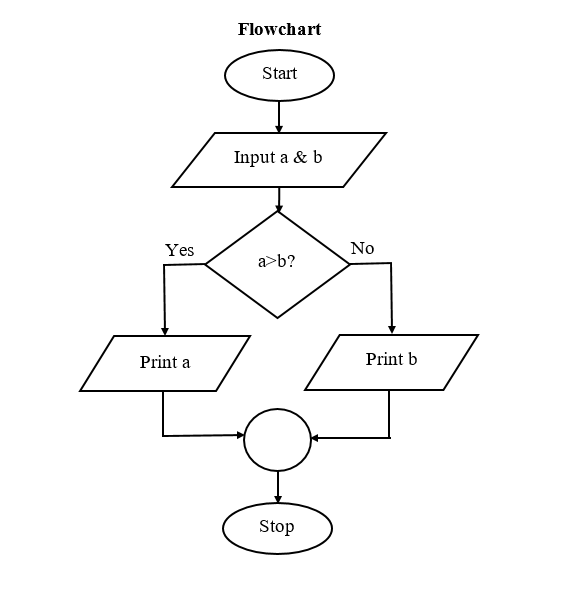5. Algorithm & flowchart for determining LCM of two integer numbers.

• Algorithm
• Step-1: Start
• Step-2: Input two numbers in variable a & b
• Step-3: If (a>b),then l=a, Otherwise l=b
• Step-4: If((l mod a=0) AND (l mod b=0)), then go to step-5,Otherwise go to step-6
• Step-5: Print value of l and go to step-7
• Step-6: l=l+1 and again go to step-4
• Step-7: Stop

Flowchart:6. Algorithm & flowchart for determining GCD of two integer numbers.

• Algorithm
• Step-1: Start
• Step-2: Input two numbers in variable a & b
• Step-3: If (a<b),then s=a, Otherwise s=b
• Step-4: If((a mod s=0) AND (b mod s=0)), then go to step-5,Otherwise go to step-6
• Step-5: Print value of s and go to step-7
• Step-6: s=s-1 and again go to step-4
• Step-7: Stop

Flowchart: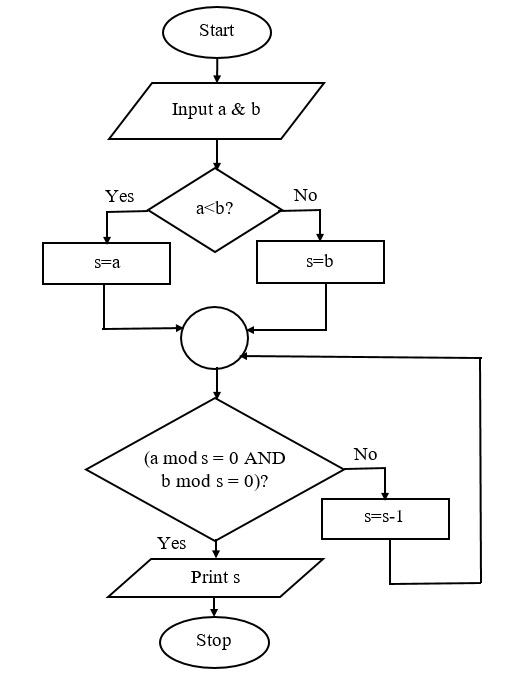7. Algorithm & flowchart for determining the smallest number among three numbers.

• Algorithm
• Step-1: Start
• Step-2: Input three numbers in variable a,b and c
• Step-3: If (a<b), then go to step-4,
•            Otherwise go to step-5
• Step-4: If (a<c), then print value of a and go to step-6
•           Otherwise print value of c and go to step-6
• Step-5: If (b<c), then print value of b,
•           Otherwise print value of c
• Step-6: Stop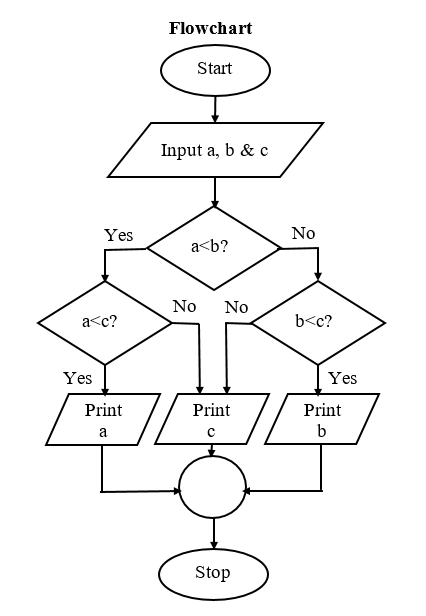8. Algorithm & flowchart for determining the largest number among three numbers.

• Algorithm
• Step-1: Start
• Step-2: Input three numbers in variable a,b and c
• Step-3: If (a>b), then go to step-4,
•            Otherwise go to step-5
• Step-4: If (a>c), then print value of a and go to step-6
•           Otherwise print value of c and go to step-6
• Step-5: If (b>c), then print value of b,
•           Otherwise print value of c
• Step-6: Stop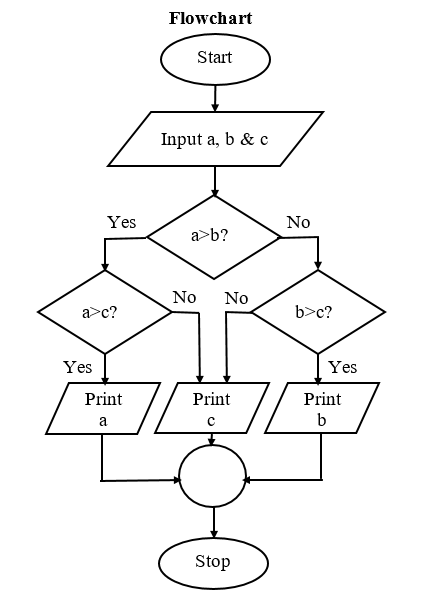Lesson Evaluation-

Knowledge Based Questions:

Comprehension Based Questions:

Creative Questions:

Multiple Choice Questions:

Written by,